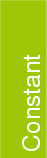## CGAL::ORIGIN

 const Origin ORIGIN;

### Definition

A symbolic constant which denotes the point at the origin. This constant is used in the conversion between points and vectors.

### Example

```  Point_2< Cartesian<Exact_NT> >  p(1.0, 1.0), q;
Vector2< Cartesian<Exact_NT> >  v;
v = p - ORIGIN;
q = ORIGIN + v;
assert( p == q );
```

### See Also

CGAL::Point_2<Kernel>
CGAL::Point_3<Kernel>